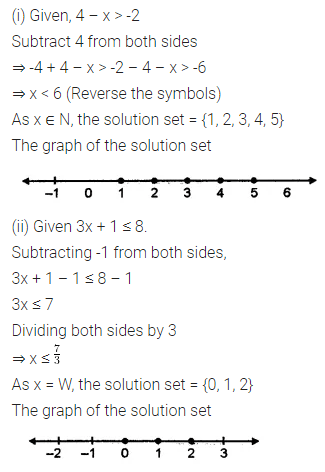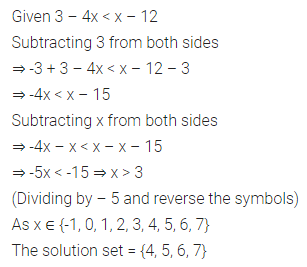# ML Aggarwal Class 7 Solutions for ICSE Maths Chapter 9 Linear Equations and Inequalities Ex 9.3

## ML Aggarwal Class 7 Solutions for ICSE Maths Chapter 9 Linear Equations and Inequalities Ex 9.3

Question 1.
If the replacement set is (- 5, – 3, – 1, 0, 1, 3, 4), find the solution set of:
(i) x < -2
(ii) x > 1
(iii) x ≥ -1
(iv) -5 < x < 3
(v) -3 ≤ x < 4
(vi) 0 ≤ x < 7.
Solution:Question 2.
Represent the following inequations graphically:
(i) x ≤ 3, x ∈ N
(ii) x < 4, x ∈ W
(iii) -2 ≤ x < 4, x ∈ I
(iv) -3 ≤ x ≤ 2, x ∈ I
Solution:Question 3.
Solve the following inequations.
(i) 4 – x > -2, x ∈ N
(ii) 3x + 1 ≤ 8, x ∈ W
Also represent their solutions on the number line.
Solution:Question 4.
Solve 3 – 4x < x – 12, x ∈ {-1, 0, 1, 2, 3, 4, 5, 6, 7}.
Solution:Question 5.
Solve -7 < 4x + 1 ≤ 23, x ∈ I.
Solution: